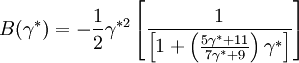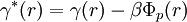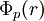Difference between revisions of "Duh Haymet"$B(\gamma^{*})= - \frac{1}{2} \gamma^{*2} \left[ \frac{1}{ \left[ 1+ \left( \frac{5\gamma^{*} +11}{7\gamma^{*} +9} \right) \gamma^{*} \right]} \right]$
where (Eq. 10)$\gamma^{*}(r) = \gamma (r) - \beta \Phi_p(r)$ where$\Phi_p (r)$ is the perturbative (attractive) part of the pair potential.